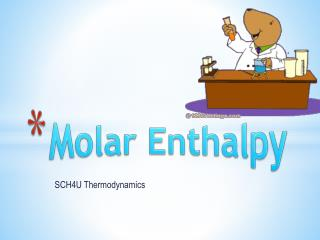# Molar Enthalpy - PowerPoint PPT PresentationDownload PresentationMolar Enthalpy

Molar Enthalpy
Download Presentation## Molar Enthalpy

- - - - - - - - - - - - - - - - - - - - - - - - - - - E N D - - - - - - - - - - - - - - - - - - - - - - - - - - -
##### Presentation Transcript

1. Molar Enthalpy SCH4U Thermodynamics

2. What is Enthalpy (H)? • All Kinetic and potential energy in a molecule Kinetic (EK) : moving e-, vibrating atoms, bond rotation Potential (EP): Bond Energy and nuclear energy Let’s Review yesterday….

3. Energy difference between the reactants and products. ΔH = Hproducts - Hreactants As long as pressure remains constant, the enthalpy change of the chemical system is equal to the flow of thermal energy in and out of the system or… ΔHsystem = l qsysteml Changes in Enthalpy, ΔH

4. The change in bond energy (ΔH) for 1 mole of a substance… Molar Enthalpy; ΔHX

5. Review: The Mole

6. Determine the ΔHcombustion for hexane if burning 30g releases 1452kJ. If the combustion of 1 mole of butane releases 2871 kJ, how much heat will be released by the combustion of 13g? Learning Check…

7. Determine the enthalpy change (ΔH) of a rxn by measuring the energy change (q) of the surroundings. The law of conservation of energy: ΔHsystem = -qsurroundings Calorimetry

8. To determine the molar enthalpy (ΔHX) during a reaction: • q = mcΔT • n = m/M or n = cv • ΔHmol = -q/n

9. Determine the ΔHcombustion for propane if 21 g of propane raises 5g of water by 50°C Learning Check…

10. If the ΔHcombsi 34.5 kJ/mol and 2.75 mol of the substance are combusted, what is the energy of the rxn? q = -nΔHmol

11. Calculate ΔH for Dissolution Reactions: An energy change called enthalpy of solution, ΔHsol, occurs when a substance dissolves in water. A student places 125g of liquid water at 24.2°C into a coffee-cup calorimeter, and then adds 10.5g of solid potassium bromide, KBr(s), also at 24.2°C. He stirs the liquid until the potassium bromide dissolves, and then determines that the temperature has changed to 21.1°C. Calculate the molar enthalpy change for this dissolution reaction, ΔHsol. Assume that the specific heat capacity, c, of the liquid in the calorimeter is the same as the specific heat capacity of water. Learning Check…one more toughy

12. Draw potential energy diagrams for each of the following: • CO2(s) + 20.3 kJ  CO2 (g) • CaCl2 (s)  CaCl2(aq) ΔHsol = -82.8 kJ • 2 Ag (s) + Cl2 (g)  2 AgCl (s) + 254 kJ Potential Energy Diagrams Practice